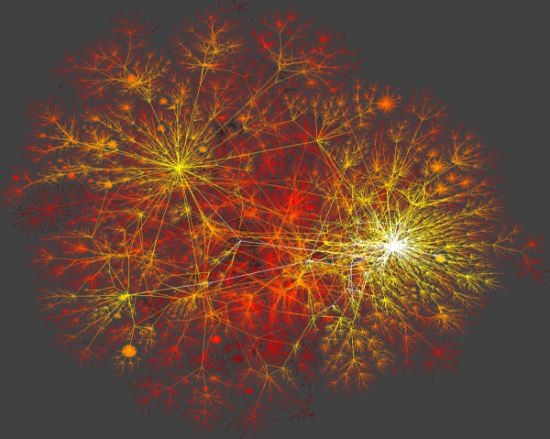# Barabasi-Albert model

Recently I have discovered an online education system Coursera. While browsing through the available courses I noticed one named "Social and Economic Networks: Models and Analysis". Sadly it was already six weeks into the eight week program, so I was not able to formally complete it. Yet the knowledge I have obtained by viewing videos of this course enabled me to prepare couple of posts for this website.

Previously, I have already written about the Erdos-Renyi and the Watts-Strogatz ("small world" networks) network formation models. This time I'll write about the Barabasi-Albert model ("scale-free" networks).

## The essence of the Barabasi-Albert model

Previously described Erdos-Renyi and Watts-Strogatz network formation models have very the in common with the real life networks. The latter is just random graph, while the former is only able to reproduce the "small world" properties. Both these models posses characteristic scale, while the real life networks do not have such. They are scaleless and posses power law degree distributions.

The power law degree distributions were reproduced by Barabasi and Albert by using the preferential attachment algorithm. In this algorithm the new nodes select old nodes, with which they will form links (edges), based on their degree (number of links the nodes has). Due to this preference for the higher degree nodes the algorithm is called preferential attachment. Due to this preference the nodes with higher degrees will further increase, while the lower degree nodes will stay with the same degree. This effect in the sociology is known as Matthew effect or "rich gets richer" effect. In the empirical data this effect is observed as power law distribution.

How to understand this effect? The internet proves to be an excellent example. There are couple of very well known and influential website on the web (Wikipedia, Google, Yahoo, BBC and etc), while there are lots of less well known (or completely unknown) websites. Those influential websites are highly cited, and thus more easily found than the ones which are less known. Thus the average internet user, then creating his own website, will surely reference the well known websites more than those which are less known. This is naturally expected not only due to the fact that he cannot know as much less known websites, but also due to the fact that majority of users see the popular website as some kind of authority figure. Consequently the already popular website will get more popular, while the less well known will remain behind them in terms of popularity. Similar situation is observed in the scientific citation networks and also in the internet connections (see the figure below).Fig 1.A macroscopic snapshot of Internet connectivity.

Taking the above into account we can mathematically express the probability that the node $$i$$ will gain another degree as

\begin{equation} p_i = \frac{k_i^\alpha}{\sum_j k_j^\alpha} . \end{equation}

In the above $$k_i$$ is a degree of the node $$i$$, $$\alpha$$ is an influence of the popularity (the larger it gets the more larger degrees are preferred), while the division by the sum over all old nodes is required to have a normalized probability.

In general case before applying the preferential attachment random connection algorithm one needs to have some set of the initial nodes. After creating this set (and edges between the nodes in it) we can apply the algorithm by creating one or more nodes at each step. It is usually required that the number of nodes created per step should be less than the size of initial set of nodes. In our applet below we present the simplest case - the initial set consists of two nodes and one node per step is being added.

## Essential properties of the Barabasi-Albert model and scale-free networks

First of all the distribution of degrees is a power law :

\begin{equation} p(k) \sim k^{-\lambda}, \end{equation}

here $$\lambda$$ is the slope of the power law function ($$\lambda=3$$, when $$\alpha=1$$).

The average path length in the scale-free networks (generated using Barabasi-Albert model) is smaller than in a case of the Watts-Strogatz model :

\begin{equation} l \sim \frac{\ln N}{\ln \ln N}. \end{equation}

Therefore it should be evident that the scale-free networks are ultra-small world networks.

And lastly, the scale-free network has a large clustering coefficient. Larger than the one observed in the Erdos-Renyi networks .

## Interactive HTML5 applet

Below you should find a HTML5 applet, which allows you to generate the scale-free networks (with different $$\alpha$$). By using left mouse click you should be able to pan the figure, while using the mouse wheel you can zoom in and out of the figure. The network can be generated manually node by node ("Add node" button) or automatically at the speed of one node per second ("Generate" button).

On the left panel we plot the scale-free graph itself, while on the right panel you can see the probability density of the network's nodes' degrees (note that both axes are logarithmic).

Note that with a large number of nodes (100 or more) the applet might free and disrupt your browser operation, thus you should handle the applet with care. If you would like to analyze or generate large network consider downloading Gephi or obtaining other similar software packages.